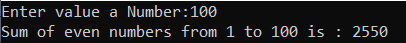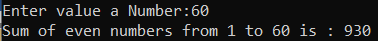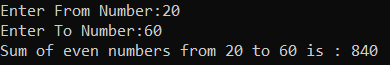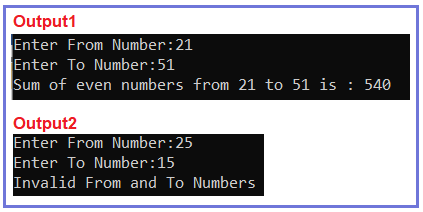# Find sum of even numbers from 1 to N in C#

## How to Find the sum of even numbers from 1 to N in C#

In this article, I am going to discuss How to find the sum of even numbers from 1 to N in C# with Examples. Please read our previous article, where we discussed How to Count the Number of Digits in a given integer number in C#. Here, in this article, first, we will discuss what are Even Numbers, then we will discuss how to find the sum of even numbers between a range of numbers and finally, we will see how to implement the sum of even number Program in C#.

###### What is an even number?

A number that is divisible by 2 and generates a remainder of 0 is called an even number. For example, 2,4,6, 8, 10, etc.

###### How to check if a number is even or not?

if(number % 2 == 0)
This is an even number.
else
This is not an even number.

##### How to find the sum of even numbers from 1 to N in C#?

Let say we want to find the sum of even number from 1 to 100, there are 50 even numbers and 50 odd numbers. This basically means every second number is an even number.
Example: 2, 4, 6, 8, etc. are even numbers and 1, 3, 5, 7, etc. are odd numbers
2 then 4 (3 is an odd number)
4 then 6 (5 is an odd number)
6 then 8 (7 is an odd number) and so on.

##### Algorithm to find the sum of even numbers

Step1: Take a variable named N and store the value of the upper limit of N.
Step2: Take one more variable named sum to store the result of the calculation.
Step3: Take a for loop with variable i which be initialized with 2(even number starts with 2) and in condition expression check for (i<=N) and in the third expression of for loop increment the value of i with 2(because every second number is odd/even).
for(int i=2;i<=N;i=i+2)
sum += i; //sum = sum + i;

##### Example: C# Program to find the sum of even number from 1 to n

The following C# Program will find the sum of even numbers from 1 to N using for loop.

```using System;
namespace DotNetTutorials
{
class SumOfEvenNumbers
{
static void Main(string[] args)
{
int sum = 0;
Console.Write("Enter value a Number:");

//2+2 = 4
//4+2 = 6
for (int i = 2; i <= Number; i += 2)
{
sum += i;
}
Console.Write(\$"Sum of even numbers from 1 to {Number} is : {sum}");
}
}
}
```
###### Output:###### Example: Using while loop

The following C# Program will find the sum of even numbers from 1 to N using the while loop.

```using System;
namespace DotNetTutorials
{
class SumOfEvenNumbers
{
static void Main(string[] args)
{
int sum = 0, i = 2;
Console.Write("Enter value a Number:");

while (i <= Number)
{
sum += i;
i += 2;
}

Console.Write(\$"Sum of even numbers from 1 to {Number} is : {sum}");
}
}
}
```
###### Output:##### Finding the sum of even numbers between a Range of Numbers:

The examples we have discussed earlier are used to find the sum of even numbers started from 1. But what if we don’t want to start from 1, instead we can give any numbers, let’s say we want to find the sum of even numbers from 50 to 100. The following C# program will ask the user to enter two numbers (the first number should be less than the second number) and then calculate the sum of even numbers.

```using System;
namespace DotNetTutorials
{
class SumOfEvenNumbers
{
static void Main(string[] args)
{
int sum = 0;
Console.Write("Enter From Number:");

Console.Write("Enter To Number:");

if(FromNumber < ToNumber)
{
for (int i = FromNumber; i <= ToNumber; i++)
{
if (i % 2 == 0)
sum += i;
}

Console.Write(\$"Sum of even numbers from {FromNumber} to {ToNumber} is : {sum}");
}
else
{
Console.Write("Invalid From and To Numbers");
}

}
}
}
```
###### Output:###### Using Function

In the below C# program, we have created one method to find the sum of even numbers between a range of numbers.

```using System;
namespace DotNetTutorials
{
class SumOfEvenNumbers
{
static void Main(string[] args)
{
int sum = 0;
Console.Write("Enter From Number:");

Console.Write("Enter To Number:");

if(FromNumber < ToNumber)
{
sum = FindSumOfEvenNumbers(sum, FromNumber, ToNumber);
Console.Write(\$"Sum of even numbers from {FromNumber} to {ToNumber} is : {sum}");
}
else
{
Console.Write("Invalid From and To Numbers");
}

}

private static int FindSumOfEvenNumbers(int sum, int FromNumber, int ToNumber)
{
for (int i = FromNumber; i <= ToNumber; i++)
{
if (i % 2 == 0)
sum += i;
}

return sum;
}
}
}
```
###### Output:In the next article, I am going to discuss How to Find the sum of odd numbers from 1 to N in C# with Examples. Here, in this article, I try to explain How to Find the sum of even numbers from 1 to N in C# with Examples and I hope you enjoy this How to Find the sum of even numbers from 1 to N article.